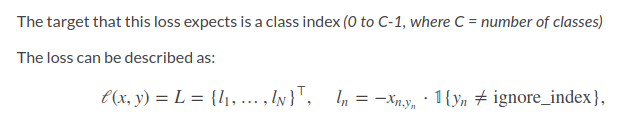# Why there is no LOG operator in implementation of torch.nn.NLLLoss

As stated in pytorch documentation, `NLLLoss` is defined as:I found there is no `log` operator in NLLLoss which is different from what I saw in eq.80 in chaper3 of book Neural Networks and Deep Learning.
Also I found in documentation it explains `torch.nn.CrossEntropyLoss` as a combination of `LogSoftMax` and `NLLLoss` ,which is also different from the implemention of `SoftmaxWithLoss` in Caffe as a combination of Softmax and multinomial logistic loss.
I wonder why there is no log operator in `torch.nn.NLLLoss` and we have to stack a `LogSoftMax` layer before `NLLLoss` layer if we want to use `NLLLoss` ? Why not put log operator in `NLLLoss` and take the result of `softmax` as input?

thanks.

Separating log and softmax might lead to numerical instability which is why you should use `log_softmax` as one function.
For `NLLLoss` you need to pass `log_softmax(x)` into the criterion. It you prefer to handle raw logits, you can use `CrossEntropyLoss`, which adds `LogSoftmax` by itself.

Oh, thanks, what you said helped me a lot. I always think wrongly that it is only separating softmax and NLLLoss than will lead to numerical instability.
I also found the reason why torch combines log and softmax in documentation of `torch.nn.functional.log_softmax`.

#### torch.nn.functional.log_softmax(input, dim=None, _stacklevel=3)

Applies a softmax followed by a logarithm.
While mathematically equivalent to log(softmax(x)), doing these two operations separately is `slower, and numerically unstable`. This function uses an alternative formulation to compute the output and gradient correctly.

So would you please explain why separating softmax and log is numerically unstable ? giving me some materials about that is also welcome.

Thank you.

You can find some information about the stability here.

1 Like

I have read the post. My understanding is that the numerical instability comes from the implementation of softmax and there are two alternatives to solve the problem, `log-sum-exp` is one choice that pytorch used. We can also seperate log and softmax but implement softmax in a more stable way by shifting the exponment.
But considering we need to calculate logarithm of softmax output in the NLLLoss, using the trick `log-sum-exp` will be faster. So we just early calculate logarithm of softmax output that should been calculated in NLLLoss.
Please let me know if I am wrong.

This is at least how I understand it.
You can have a look at the log-sum-exp usage here.